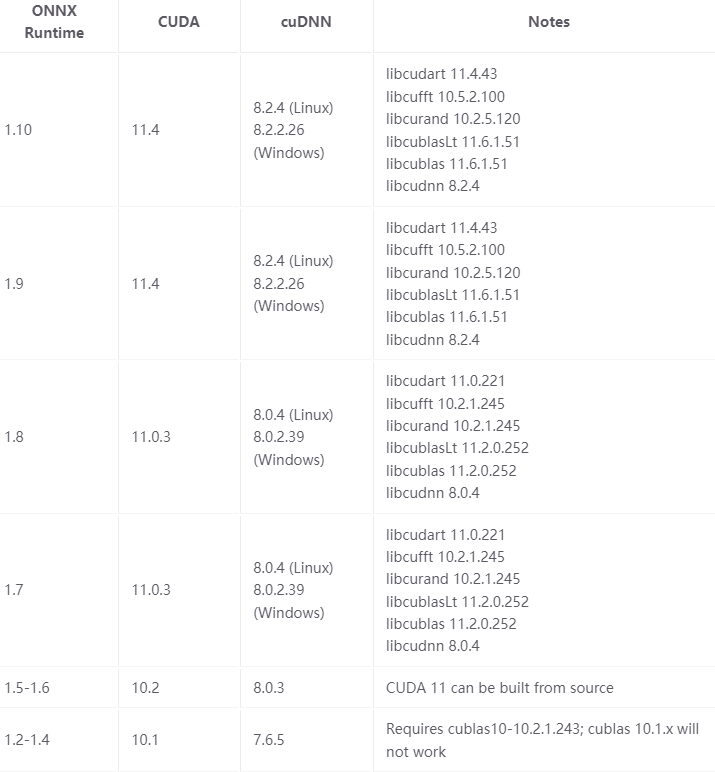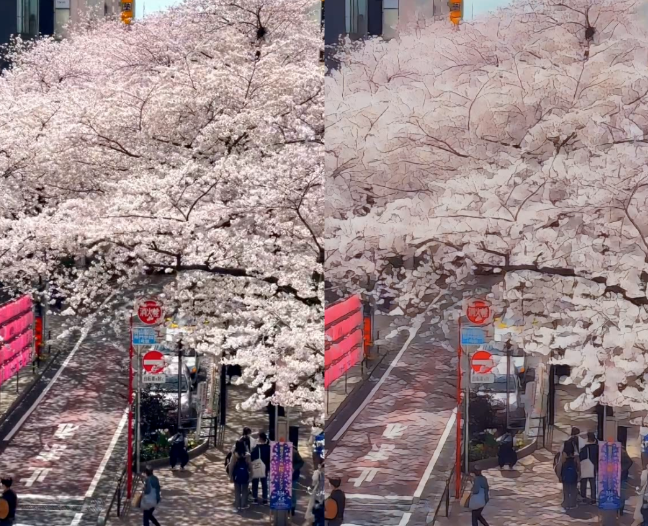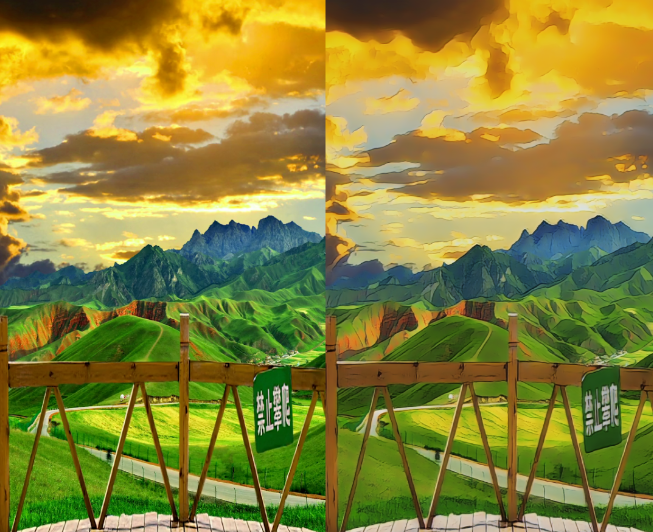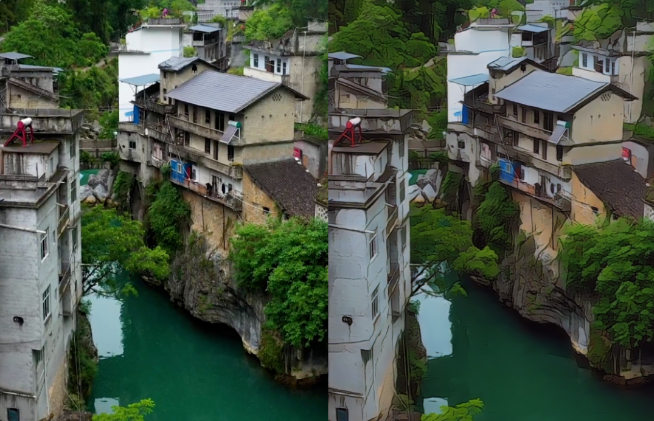# 最近几个月，毒教材被曝光引发争议，那些编写度教材的人着实可恶。咱程序员也没有手绘插画能力，但咱可以借助强大的深度学习模型将视频转动漫。所以今天的目标是让任何具有python语言基本能力的程序员，实现短视频转动漫效果。# 一、思路流程

1. 读取视频帧
2. 将每一帧图像转为动漫帧
3. 将转换后的动漫帧转为视频

# 二、图像转动漫

```pip install onnxruntime
# 源码及Python资料教程、电子书领取群 279199867```

`pip install onnxruntime-gpu`

onnxruntime-gpu的版本跟CUDA有关联，具体对应关系如下：```import onnxruntime as rt
sess = rt.InferenceSession(MODEL_PATH)
inp_name = sess.get_inputs().name
out = sess.run(None, {inp_name: inp_image})```

```import cv2
import numpy as np
import onnxruntime as rt

# MODEL = "models/anime_1.onnx"
MODEL = "models/anime_2.onnx"

sess = rt.InferenceSession(MODEL)
inp_name = sess.get_inputs().name

def infer(rgb):
rgb = np.expand_dims(rgb, 0)
rgb = rgb *  2.0 / 255.0 - 1
rgb =  rgb.astype(np.float32)
out = sess.run(None, {inp_name: rgb})
out = out
out = (out+1)/2*255
out = np.clip(out, 0, 255).astype(np.uint8)
return out

def preprocess(rgb):
h,w,__ = rgb.shape
N = 2**3
if h%N!=0:
if w%2!=0:# 三、视频帧读取与视频帧写入

```import cv2
from tqdm import tqdm

WRITER = None
def write_frame(frame, out_path, fps=30):
global WRITER
if WRITER is None:
size = frame.shape[0:2][::-1]
WRITER = cv2.VideoWriter(
out_path,
cv2.VideoWriter_fourcc(*'mp4v'),  # 编码器
fps,
size)
WRITER.write(frame)

def extract_frames(video_path, callback):
video = cv2.VideoCapture(video_path)
num_frames = int(video.get(cv2.CAP_PROP_FRAME_COUNT))
for _ in tqdm(range(num_frames)):